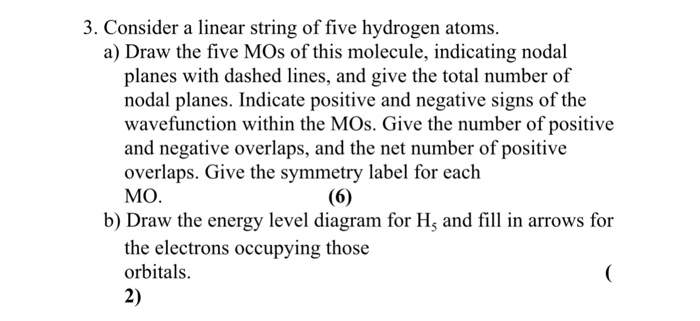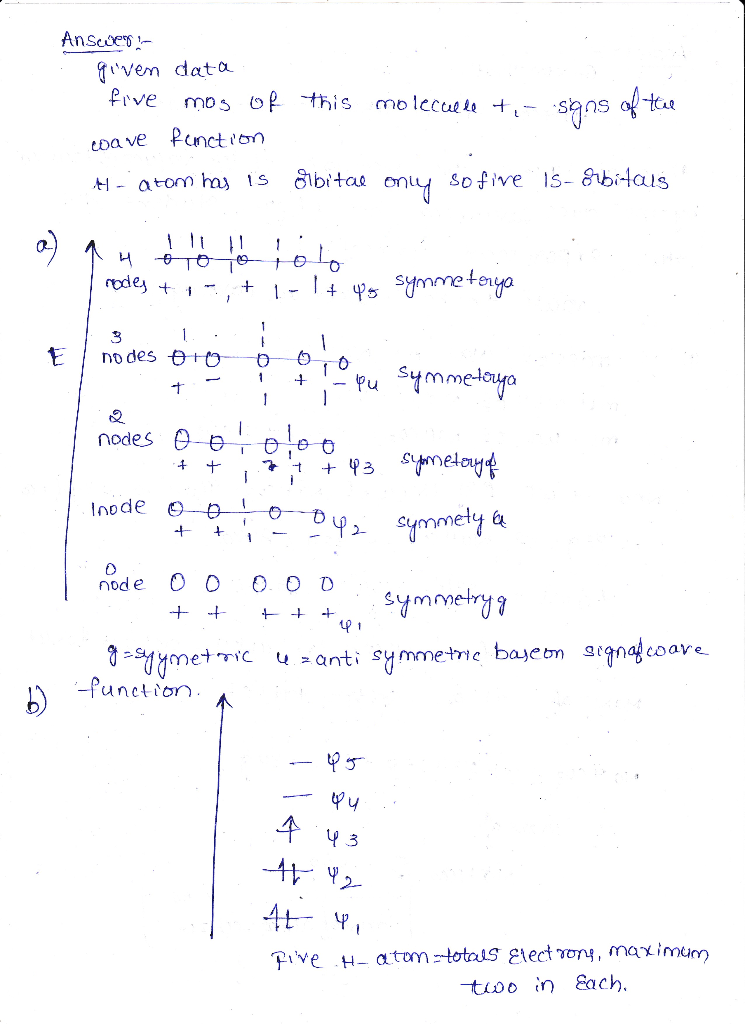# Can you explain how you do each step. i dont know how to do it.

can you explain how you do each step. i dont know how to do it.3. Consider a linear string of five hydrogen atoms. a) Draw the five MOs of this molecule, indicating nodal planes with dashed lines, and give the total number of nodal planes. Indicate positive and negative signs of the wavefunction within the MOs. Give the number of positive and negative overlaps, and the net number of positive overlaps. Give the symmetry label for each MO b) Draw the energy level diagram for Hs and fill in arrows for the electrons occupying those orbitals. 2)##### Add Answer of: Can you explain how you do each step. i dont know how to do it.
More Homework Help Questions Additional questions in this topic.